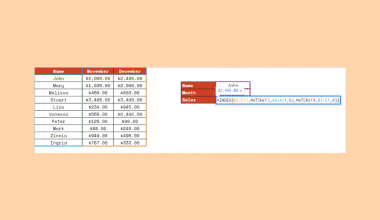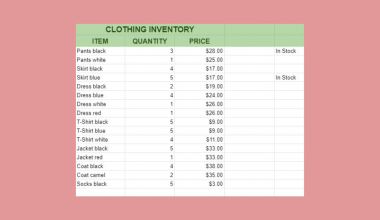# How to Use the ERF Function in Google Sheets

The `ERF` function in Google Sheets calculates for the Gauss error function of a value or an interval of values.

In other words, it returns the error function integrated between lower and upper limits or even just a lower limit. It’s a statistical tool that you encounter when integrating the normal distribution. The number generated represents how well your model fits the data. A lower number means you have less error.

`ERF` is defined as follows:For a random variable normally distributed with a mean of 0 and a variance of 1/√2, ERF(x) describes the probability of the variable falling in the given range.

This is helpful for various complex problem-solving scenarios like determining how much of a nominal 100g is an outlier. In particular, if you’re selling milk with 100g per container and you know that the standard deviation is 1, you should be able to find out how many of the items are [99g, 101g].

Now, let’s learn how to write the `ERF` function in Google Sheets.

## The Anatomy of the ERF Function in Google Sheets

The syntax (the way we write) of the `ERF` function goes as follows:

`=ERF(lower_bound, [upper_bound])`

Let’s dissect this thing and understand what each of these terms means:

• = the equal sign is just how we start any function in Google Sheets.
• `ERF` () is our Gauss error function.
• lower_bound is the lower boundary for the integral.
• [upper_bound] is the upper boundary for the integral. Note that you can omit this parameter from the function. This would assume that the integration is between 0 and the lower_bound.

Note that you will get the #VALUE! error value if your lower_bound and upper_bound are non-numeric.

## Real Example of Using the ERF Function in Google Sheets

Take a look at some numbers I’m presenting to see how an ERT function will look like in Google Sheets.In the first table, we see that only the lower limit is used in the `ERF` function. As mentioned, this assumes 0 as its pair. Meanwhile, in the second table, the upper limit is given.

Besides the negative and positive signs, the result should be the same for (-1,0) and (0,1) since their distance on a distribution table is the same.

At this point, I’ll show you how you can do this yourself with comprehensive steps.

## How to Use the ERF Function in Google Sheets

1. First, select the cell where you wish to put the result of your `ERF` function. In this case, that’s C3.2. Next, enter the equal sign ‘=’ to begin the function. Then, follow it with the name of the function, which is ‘`ERF`’ (or ‘`ERF`’, both should work). An auto-suggest box should appear. There, you can click on the ERT option or type an open parenthesis ‘(‘.  You can read the pop-out with more information about the function.3. Now, enter the number that serves as your lower_bound. In this case, we select the cell where the data is entered prior, which is A34. At this point, we can either select an upper limit or leave none to use the default pair of 0. To do the latter, simply enter a close parenthesis ‘)’ and select Enter on your keyboard.5. To add an upper limit, separate the parameter with a comma (,). Then, enter the number you intend. In this case, select the cell where the data is entered prior, which is B3. Finally, close the function with a close parenthesis ‘)’ and select Enter on your keyboard.That’s it! You’ve just learned how to use the ERF function in Google Sheets.

What is `ERFC`?

`ERFC` stands for ‘complementary error function’ and gives out the value of 1.0 -erf(x). Its syntax is “ERFC(x)” where x is the number to calculate the complementary Gauss error function. For larger values of x, it is useful in place of the `ERF` function.

How do I use `ERF` in Excel?

`ERF` is used the same in Google Sheets and Excel. With that, simply follow the instructions in this article to use `ERF` in Excel. Note that in Excel 2007, you will get the #NUM! error value if you enter a negative value in the parameters. Calculating this function for both positive and negative ranges has been possible since Excel 2010.

What is `ERF` in Heat Transfer?

The `ERF` function is commonly used in engineering, particularly in heat conduction problems. The formula is as follows:Here, erf(x) is the Gauss error function, t is time, and x is a complex number.

Make sure to explore the other Google Sheets functions available that can help you with mathematics and data manipulation. Also, comment down below if you have any more inquiries and if you’d like to give suggestions on what topic we should write about next. Make sure to subscribe to stay updated!Our goal this year is to create lots of rich, bite-sized tutorials for Google Sheets users like you. If you liked this one, you'll love what we are working on! Readers receive ✨ early access ✨ to new content.

##### You May Also Like## How to Create a Pareto Chart in Google Sheets

A Pareto chart in Google Sheets is a kind of combo chart that has both bars and lines…## How To Use INDEX and MATCH Together in Google Sheets

The INDEX and MATCH together in Google Sheets come useful if you want to perform lookups. Using the…## How to Use CONVERT Function in Google Sheets

The CONVERT function in Google Sheets is useful if you want to convert a number in one measurement…## How to Protect a Range of Cells in Google Sheets

In this tutorial, we’ll learn how to protect a range of cells in Google Sheets. We’ll see different…